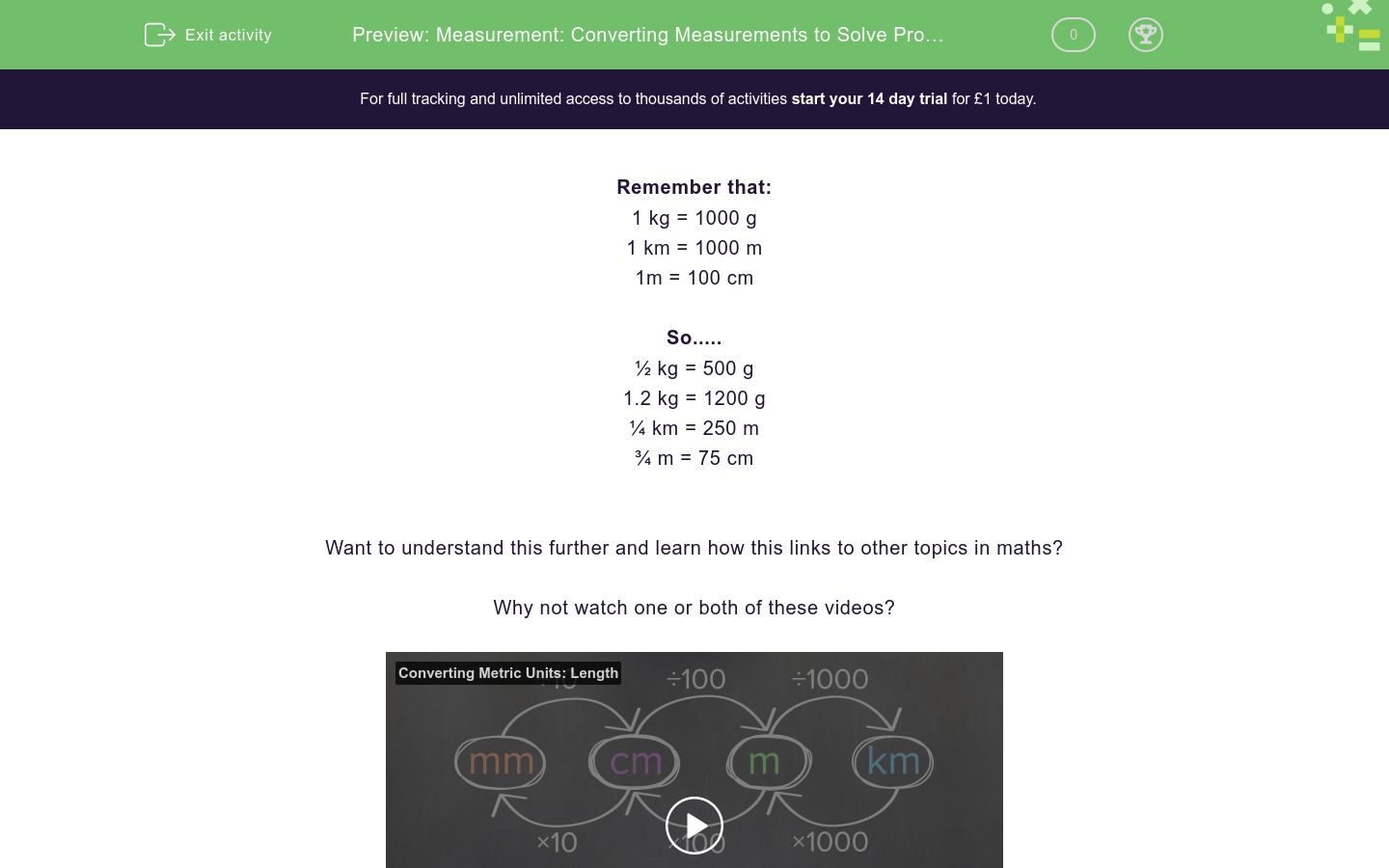# Measurement: Converting Measurements to Solve Problems

In this worksheet, students convert between metric measurements and answer measuring problems.Key stage:  KS 2

Curriculum topic:   Measurement

Curriculum subtopic:   Convert Between Metric Measurements

Difficulty level:### QUESTION 1 of 10

Remember that:

1 kg = 1000 g

1 km = 1000 m

1m = 100 cm

So.....

½ kg = 500 g

1.2 kg = 1200 g

¼ km = 250 m

¾ m = 75 cm

Want to understand this further and learn how this links to other topics in maths?

Why not watch one or both of these videos?

A tree grows to a height of 5.5 metres.

Write this height in cm.

(just write the number)

Who is taller?

Jack is 1.2 m tall

Jill is 118 cm tall

Work out ½ kg + ¼ kg, giving your answer in grams.

(just write the number)

A 1 kg bag of flour is opened and Ben uses 450 g to bake a cake.

How much flour is left in the bag?

450 g

550 g

0.55 kg

0.055 kg

A cat has a mass of 3.5 kg

Write this mass in g.

(just write the number)

A cat has a mass of 3.5 kg and a dog has a mass of 5¼ kg

Write their total mass in kg.

(just write the number)

A sunflower is 2.4 m tall.

In one week, it grows 50 cm.

What is its height at the end of this week in metres.

(just write the number)

Work out 1 km - 50 m.

(just write the number)

A box of chocolates has a mass of 1.4 kg.

700 g is eaten after dinner.

Write down the mass of chocolates which remain in kg.

(just write the number)

I cycle 750 m and then walk 1.5 km.

How far have I traveled altogether in metres?

(just write the number)

• Question 1

A tree grows to a height of 5.5 metres.

Write this height in cm.

(just write the number)

550
EDDIE SAYS
1m = 100 cm 5.5m = 5.5 x 100 = 550 cm
• Question 2

Who is taller?

Jack is 1.2 m tall

Jill is 118 cm tall

Jack
EDDIE SAYS
1m = 100 cm 1.2m = 1.2 x 100 = 120 cm Jack is 120 cm and Jill is 118 cm, so Jack is taller.
• Question 3

Work out ½ kg + ¼ kg, giving your answer in grams.

(just write the number)

750
EDDIE SAYS
½ kg = 500 g 1/4 kg = 1000/4 = 250 g 500 + 250 = 750 g
• Question 4

A 1 kg bag of flour is opened and Ben uses 450 g to bake a cake.

How much flour is left in the bag?

550 g
0.55 kg
EDDIE SAYS
1 kg = 1000 g 1000 - 450 = 550 g 550 divided by 1000 = 0.55 kg
• Question 5

A cat has a mass of 3.5 kg

Write this mass in g.

(just write the number)

3500
EDDIE SAYS
1 kg = 1000 g 3.5 kg = 3.5 x 1000 = 3500 g
• Question 6

A cat has a mass of 3.5 kg and a dog has a mass of 5¼ kg

Write their total mass in kg.

(just write the number)

8.75
EDDIE SAYS
1 kg = 1000 g 1/4 kg = 1000/4 = 250 g 5 kg = 5 x 1000 = 5000 g + 250 g = 5250g 5250 divided by 1000 = 5.25 kg 3.5 kg + 5.25 kg = 8.75 kg
• Question 7

A sunflower is 2.4 m tall.

In one week, it grows 50 cm.

What is its height at the end of this week in metres.

(just write the number)

2.9
EDDIE SAYS
1m = 100 cm 2.4 m = 2.4 x 100 = 240 cm 240 + 50 = 290 cm 290 divided by 100 = 2.9 m
• Question 8

Work out 1 km - 50 m.

(just write the number)

0.95
EDDIE SAYS
1 km = 1000 m 1000 - 50 = 950 m 950 divided by 1000 = 0.95
• Question 9

A box of chocolates has a mass of 1.4 kg.

700 g is eaten after dinner.

Write down the mass of chocolates which remain in kg.

(just write the number)

0.7
EDDIE SAYS
1 kg = 1000 g 1.4 kg = 1400 g 1400 - 700 = 700 700/1000 = 0.7 kg
• Question 10

I cycle 750 m and then walk 1.5 km.

How far have I traveled altogether in metres?

(just write the number)

2250
EDDIE SAYS
1 km = 1000 m 1.5 km = 1.5 x 1000 = 1500 m 1500 + 750 = 2250 m
---- OR ----

Sign up for a £1 trial so you can track and measure your child's progress on this activity.

### What is EdPlace?

We're your National Curriculum aligned online education content provider helping each child succeed in English, maths and science from year 1 to GCSE. With an EdPlace account you’ll be able to track and measure progress, helping each child achieve their best. We build confidence and attainment by personalising each child’s learning at a level that suits them.

Get started